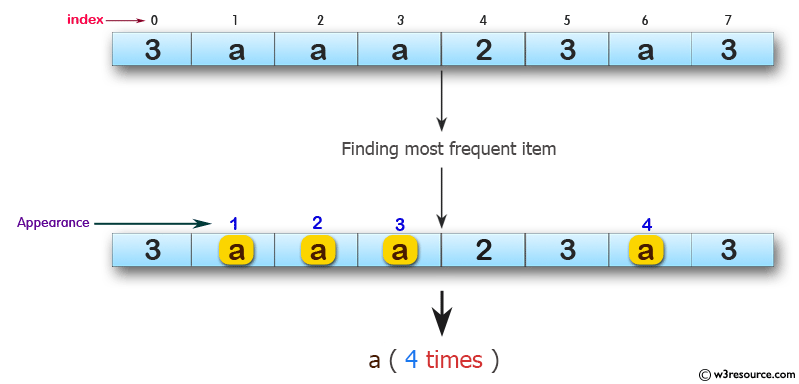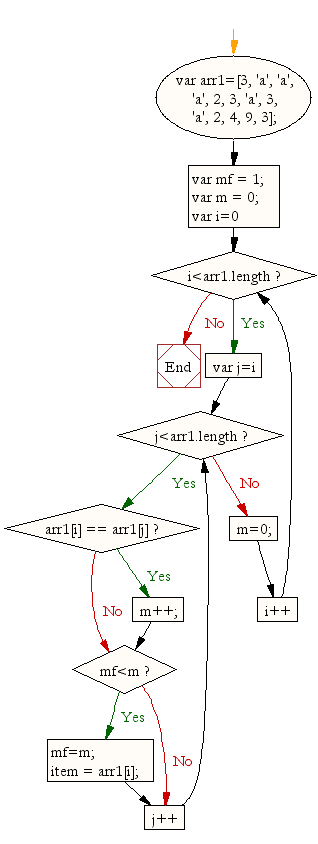# JavaScript: Find the most frequent item of an array

## JavaScript Array: Exercise-8 with Solution

Write a JavaScript program to find the most frequent item of an array.

Sample array: var arr1=[3, 'a', 'a', 'a', 2, 3, 'a', 3, 'a', 2, 4, 9, 3];
Sample Output: a ( 5 times )

Pictorial Presentation:Sample Solution:

HTML Code:

``````<!DOCTYPE html>
<html>
<meta charset=utf-8 />
<title>Write a JavaScript program to find the most frequent item of an array. - w3resource</title>
<body>
</body>
</html>
```
```

JavaScript Code:

``````var arr1=[3, 'a', 'a', 'a', 2, 3, 'a', 3, 'a', 2, 4, 9, 3];
var mf = 1;
var m = 0;
var item;
for (var i=0; i<arr1.length; i++)
{
for (var j=i; j<arr1.length; j++)
{
if (arr1[i] == arr1[j])
m++;
if (mf<m)
{
mf=m;
item = arr1[i];
}
}
m=0;
}
console.log(item+" ( " +mf +" times ) ") ;
```
```

Sample Output:

```a ( 5 times )
```

Flowchart:ES6 Version:

``````const arr1=[3, 'a', 'a', 'a', 2, 3, 'a', 3, 'a', 2, 4, 9, 3];
let mf = 1;
let m = 0;
let item;
for (let i=0; i<arr1.length; i++)
{
for (let j=i; j<arr1.length; j++)
{
if (arr1[i] == arr1[j])
m++;
if (mf<m)
{
mf=m;
item = arr1[i];
}
}
m=0;
}
console.log(`\${item} ( \${mf} times ) `) ;
```
```

Live Demo:

See the Pen JavaScript - Find the most frequent item of an array- array-ex- 8 by w3resource (@w3resource) on CodePen.

Improve this sample solution and post your code through Disqus

What is the difficulty level of this exercise?

﻿

## JavaScript: Tips of the Day

JavaScript: Shorten an array using its length property

A great way of shortening an array is by redefining its length property.

```let array = [0, 1, 2, 3, 4, 5, 6, 6, 8, 9]
array.length = 4
// Result: [0, 1, 2, 3]
```

Important to know though is that this is a destructive way of changing the array. This means you lose all the other values that used to be in the array.

Ref: https://bit.ly/2LBj213